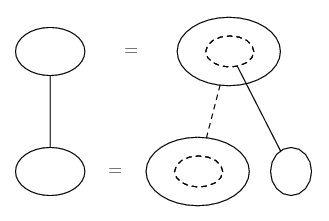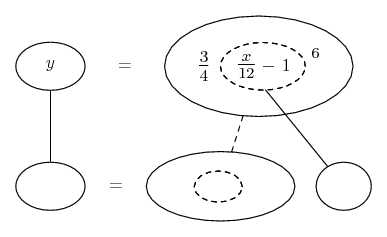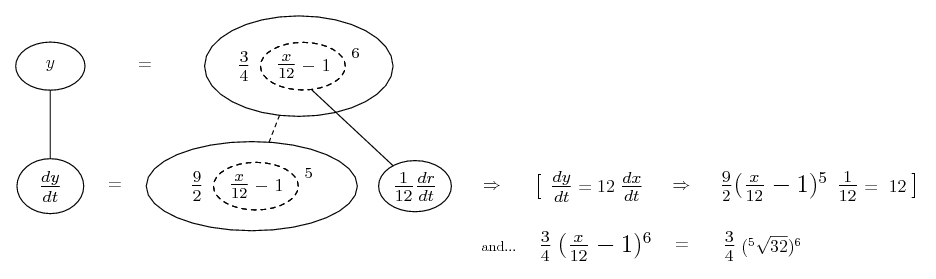# Thread: Connected rate of change

1. ## Connected rate of change

Two variables, x and y, are related by the equation

$\displaystyle y=\frac{3}{4}(\frac{x}{12}-1)^6.$

Given that both x and y vary with time, find the value of y when the rate of change of y is 12 times the rate of change of x.

2.Originally Posted by PunchTwo variables, x and y, are related by the equation

$\displaystyle y=\frac{3}{4}(\frac{x}{12}-1)^6.$

Given that both x and y vary with time, find the value of y when the rate of change of y is 12 times the rate of change of x.
take the derivative w/r to time

use the given condition $\displaystyle \frac{dy}{dt} = 12 \frac{dx}{dt}$

determine the value of x ... then determine y.

3. And, just in case a picture helps fill out the chain rule...... where straight continuous lines differentiate downwards (integrate up) with respect to the main variable (in this case time), and the straight dashed line similarly but with respect to the dashed balloon expression (the inner function of the composite which is subject to the chain rule).

Starting then with...Spoiler:_________________________________________
Don't integrate - balloontegrate!

Balloon Calculus; standard integrals, derivatives and methods

Balloon Calculus Drawing with LaTeX and Asymptote!

4. $\displaystyle y = \frac{3}{4}\left(\frac{x}{12} - 1\right)^6$

$\displaystyle \frac{dy}{dt} = \frac{9}{2}\left(\frac{x}{12} - 1\right)^5 \cdot \frac{1}{12} \cdot \frac{dx}{dt}$

$\displaystyle 12 \cdot \frac{dx}{dt} = \frac{9}{2}\left(\frac{x}{12} - 1\right)^5 \cdot \frac{1}{12} \cdot \frac{dx}{dt}$

assuming $\displaystyle \frac{dx}{dt} \ne 0$ ...

$\displaystyle 12 = \frac{9}{2}\left(\frac{x}{12} - 1\right)^5 \cdot \frac{1}{12}$

$\displaystyle 12 = \frac{3}{8}\left(\frac{x}{12} - 1\right)^5$

$\displaystyle 32 = \left(\frac{x}{12} - 1\right)^5$

$\displaystyle 2^5 = \left(\frac{x}{12} - 1\right)^5$

$\displaystyle x = 36$

calculate the value of y from the original equation.

5.Originally Posted by PunchTwo variables, x and y, are related by the equation

$\displaystyle y=\frac{3}{4}(\frac{x}{12}-1)^6.$

Given that both x and y vary with time, find the value of y when the rate of change of y is 12 times the rate of change of x.
So would I be right to say that from "the rate of change of y is 12 times the rate of change of x" $\displaystyle \frac{dy}{dx}=12$?

6. if $\displaystyle \frac{dy}{dt} = 12 \cdot \frac{dx}{dt}$ , then $\displaystyle \frac{dy}{dx} = 12$ because ...

$\displaystyle \frac{\frac{dy}{dt}}{\frac{dx}{dt}} = \frac{dy}{dx}$

7. I understood this part but... what I was trying to clarify was... From the sentence "find the value of y when the rate of change of y is 12 times the rate of change of x.", What would I be able to say from the sentence?

#### Search Tags

change, connected, rate